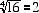Quandaries and Queries I understand that the square root is defined to be positive only. Does the same rule apply to other even-numbered roots, such as the 4th root, or the 6th root, etc.? Hi,Take a look at the answer that we gave to Frank who asked a similar question. The answer for even-numbered roots is the same as we noted there. If you asked me for the fourth root of 16 I would say it is 2 although some older books might give 2 and -2. If you are using the surd notation then the answer is 2.Harley Go to Math Central